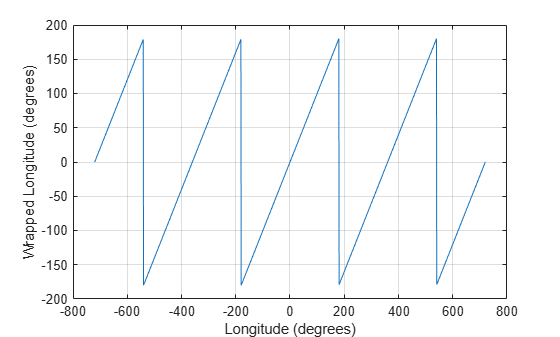wrapTo180

Wrap angle in degrees to [-180 180]

Description

example

lonWrapped = wrapTo180(lon) wraps angles in lon, in degrees, to the interval [–180, 180] such that 180 maps to 180 and –180 maps to –180. In general, odd, positive multiples of 180 map to 180 and odd, negative multiples of 180 map to –180.

Examples

collapse all

Specify a short list of longitudes to wrap.

lon = [-400 -190 -180 -175 175 180 190 380];

Wrap the longitudes to the range [-180, 180] degrees.

lonWrapped = wrapTo180(lon)
lonWrapped = 1×8

-40   170  -180  -175   175   180  -170    20

Specify a second list of longitudes that are sampled over a large range of angles. Wrap the longitudes.

lon2 = -720:720;
lon2Wrapped = wrapTo180(lon2);

Plot the wrapped longitudes. The wrapped longitudes stay in the range [-180, 180] degrees.

plot(lon2,lon2Wrapped)
xlabel("Longitude (degrees)")
ylabel("Wrapped Longitude (degrees)")
grid onInput Arguments

collapse all

Angles, specified as a numeric vector.

Data Types: single | double | int8 | int16 | int32 | int64 | uint8 | uint16 | uint32 | uint64 | logical

Output Arguments

collapse all

Wrapped angles, specified as a numeric vector with values in the range [–180, 180].

Extended Capabilities

C/C++ Code GenerationGenerate C and C++ code using MATLAB® Coder™.